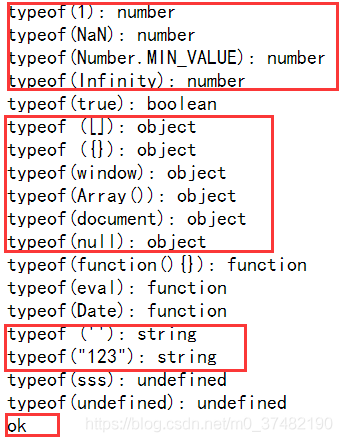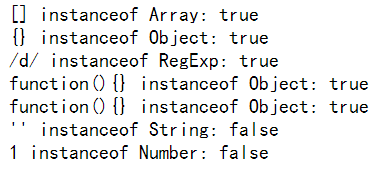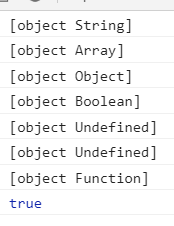# JavaScript summary: the difference between typeof and instanceof, and Object.prototype.toString() method

In my previous blog, I introduced basic data type and reference data type: basic type is a simple data segment stored in stack memory, that is, a single literal value; reference data type refers to an object composed of multiple values.

Typeof is an operator used to detect variable data types, mainly used to detect basic data types. It can determine whether a variable is a string, a numeric value, a Boolean value or undefined. However, if the detected variable is a reference data type, it can return object or function, but it can’t tell in detail whether it is array or regexp.

The main purpose of nstanceof is to detect reference types.

Let’s take a closer look

The difference between typeof and instanceof:

1.typeof：

Typeof is a unary operation, which can be of any type before an operand.

It is mainly used to determine whether the data is the basic data type:
string, number, object, null, undefined and Boolean, but it is unable to determine the function, array and regexp

the return value is a string indicating the type of the operand.

only a few results can be returned, such as number, undefined, and Boolean.

We can even use typeof to determine whether a variable exists,
such as if (typeof a = = undefined){ document.write (“OK”);}, and you don’t need to use if (a), because if a doesn’t exist (undeclared), there will be an error. For special objects such as array and null, typeof will always return objects, which is the limitation of typeof.

Take a look at the code example:

``````<!DOCTYPE html>
<html>
<head>
<meta http-equiv="Content-Type" content="text/html; charset=utf-8" />
<script language="javascript" type="text/javascript">
document.write ("typeof(1): "+typeof(1)+"<br>");
document.write ("typeof(NaN): "+typeof(NaN)+"<br>");
document.write ("typeof(Number.MIN_VALUE): "+typeof(Number.MIN_VALUE)+"<br>");
document.write ("typeof(Infinity): "+typeof(Infinity)+"<br>");
document.write ("typeof(true): "+typeof(true)+"<br>");
document.write ("typeof ([]): "+typeof([])+"<br>");
document.write ("typeof ({}): "+typeof({})+"<br>");
document.write ("typeof(window): "+typeof(window)+"<br>");
document.write ("typeof(Array()): "+typeof(new Array())+"<br>");
document.write ("typeof(document): "+typeof(document)+"<br>");
document.write ("typeof(null): "+typeof(null)+"<br>");
document.write ("typeof(function(){}): "+typeof(function(){})+"<br>");
document.write ("typeof(eval): "+typeof(eval)+"<br>");
document.write ("typeof(Date): "+typeof(Date)+"<br>");
document.write ("typeof (''): "+typeof('')+"<br>");
document.write ("typeof(\"123\"): "+typeof("123")+"<br>");
document.write ("typeof(sss): "+typeof(sss)+"<br>");
document.write ("typeof(undefined): "+typeof(undefined)+"<br>");
if(typeof a=="undefined"){document.write ("ok");}
</script>
<title>javascript</title>
</head>
<body>
</body>
</html>
``````

Running results:2.instanceof：

The main purpose of instanceof is to detect the reference type. The return value of is only true and false </ font>, which can be used to determine whether the prototype attribute of a constructor exists in the prototype chain of another object to be detected. Here we can ignore the prototype first

See the following code example:

``````<!DOCTYPE html>
<html>
<head>
<meta http-equiv="Content-Type" content="text/html; charset=utf-8" />
<script language="javascript" type="text/javascript">
document.write ("[] instanceof Array: " + ([] instanceof Array )+ "<br>");
document.write ("{} instanceof Object: " + ({} instanceof Object )+ "<br>");
document.write ("/\d/ instanceof RegExp: " +( /\d/ instanceof RegExp) + "<br>");
document.write ("function(){} instanceof Object: " + (function(){} instanceof Object) +"<br>");
document.write ("function(){} instanceof Object: " + (function(){} instanceof Function) +"<br>");
document.write ("'' instanceof String: " + ('' instanceof String) +"<br>");
document.write ("1 instanceof Number: "+ (1 instanceof Number) +"<br>");
</script>
<title>javascript类型测试</title>
</head>
<body>
</body>
</html>
``````

The running results are as follows:For example, we need to determine whether a is an instance of B?You can use a instance of B?Alert (“true”): Alert (“false”); to judge

Instanceof is used to determine whether a variable is an instance of an object
for example, var a = new array(); alert (a instanceof array); will return true
and alert (a instanceof object) will return true;
this is because array is a subclass of object.

When it comes to instanceof, we need to insert one more problem, which is the arguments of function. We may all think that arguments is an array, but if we use instanceof to test, we will find that arguments is not an array object, although it looks very similar.

In addition:

Test var a = new array(); if (a instance of object) alert (‘y ‘); else alert (‘n’);
get ‘y’

But if (window instance of object) alert (‘y ‘); else alert (‘n’);
get ‘n’

Therefore, the object of instanceof test here refers to the object in JS syntax, not the DOM model object.

There are some differences when using typeof
alert (typeof (window)) will get object

three Object.prototype.toString

In many cases, we can use the instanceof operator or the constructor property of the object to detect whether the object is an array. For example, many JavaScript frameworks use these two methods to determine whether an object is an array type. But detecting arrays in cross frame pages fails. The reason is that arrays created in different iframes do not share their prototype properties with each other

``````    <script>
window.onload=function(){
var iframe_arr=new window.frames.Array;
alert(iframe_arr instanceof Array); // false
alert(iframe_arr.constructor == Array); // false
}
</script>
``````

The tostring() method is called across the prototype chain Object.prototype.toString (), which can solve the above cross framework problem.
This is a primitive prototype extension function of an object, which is used to distinguish data types accurately
when Object.prototype.toString (o) After execution, the following steps are performed:

1) Get the class property of object o.
2) connection string “[object” + result (1) + “]
3) return result

Object.prototype.toString . call ([]);// returns “[object array]”
0 Object.prototype.toString . call (/ reg/Ig);// returns “[object regexp]”

And we will find that it is not accurate to judge the data type by typeof. For example, the return values of array, regular, date and object typeof are all objects, which will cause some errors.
Therefore, on the basis of type of judgment, we need to use Object.prototype.toString Method to further determine the data type.

Let’s look at the code:

``````<script language="javascript" type="text/javascript">
var type=Object.prototype.toString
console.log(type.call(''));//object String
console.log(type.call([]));//object Array
console.log(type.call({}));//object Object
console.log(type.call(false));//object Boolean
console.log(type.call());//object Null
console.log(type.call(undefined));//object Undefined
console.log(type.call(function(){}));//object Function
console.log(type.call('')=="[object String]");//true
</script>
``````

Running results:To sum up:

Both typeof and instanceof are operators used to detect variable types. The difference between them is that

Typeof is used to judge the basic type of variable; instanceof is used to judge the type of object;

Call the tostring() method across the prototype chain: Object.prototype.toString (), which can solve the cross frame problem.
This is a primitive prototype extension function of the object, which can be used to accurately distinguish data types